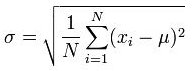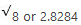Altair® Panopticon

# Stdevp

The Population Standard Deviation of the selection.

The Stdevp deals with the complete population where as Stdev deals with a population sample only.

The formula:Sample 1:

Population: A set of data that is all inclusive.

Populations are often very large. For simplicity, imagine the following as an example:

12, 6, 12

## Computing the Stdevp

1. Determine the mean of the sample.

12+6+12/3=10

1. Square the difference between each item and the mean

(12-10)^2 =4

(6-10)^2 =16

(12-10)^2 =4

1. Calculate the average.

4+16+4/3=24/3

1. Calculate the square rootSample 2:

Given the following sample fields:

 Number Arbitrary Negative Values Positive Values One Binary Currency Decimal 1 3 -1 1 1 0 \$1.00 1.01 2 2 -2 2 1 \$10.00 2.02 3 3 -3 3 0 \$100.00 3.03 4 0 -4 4 1 \$1,000.00 4.04 5 -1 -5 5 0 \$10,000.00 5.05 6 -2 -6 6 1 -\$1.00 6.06 7 -3 -7 7 0 -\$10.00 7.07 8 0 -8 8 -\$100.00 8.08 9 0 -9 9 -\$1,000.00 9.09 10 0 -10 0 \$0.00 0.00

Sample fields

The Stdevp for each field:

 Number Arbitrary Negative Values Positive Values One Binary Currency Decimal 2.8723 16733 2.8723 2.8723 0 .4949 \$3,033.4832 2.9010

Results per field